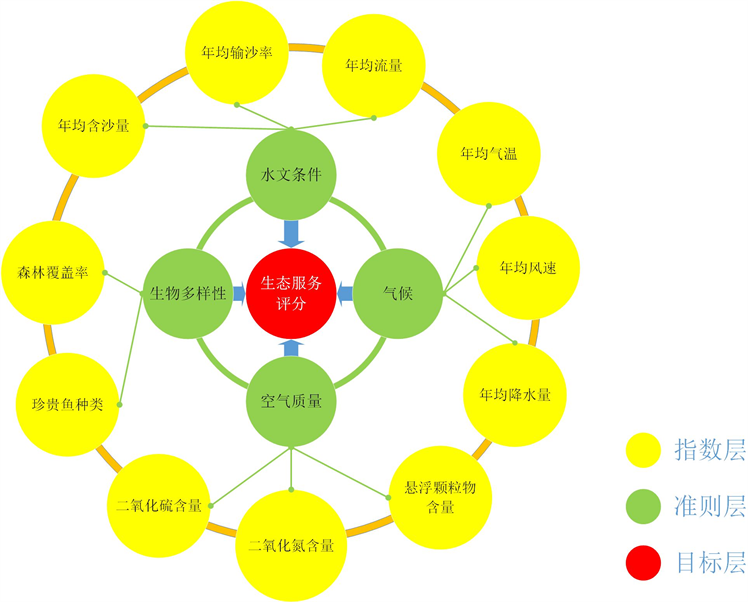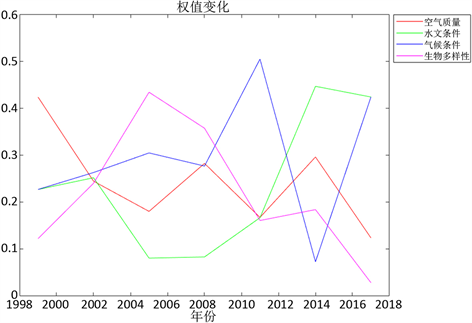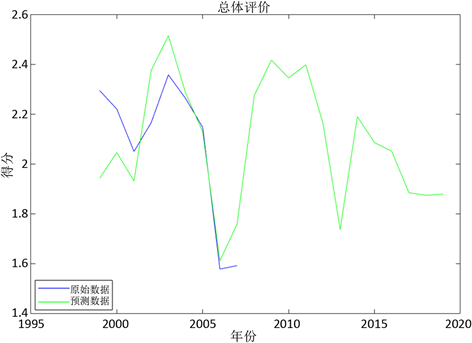﻿ 建设工程对生态环境影响的模型研究

# 建设工程对生态环境影响的模型研究Model Study on the Impact of Construct Projects on the Ecological Environment

Abstract: In order to study the impact of construct projects on the ecological environment, this paper com-bines the Analytic Hierarchy Process (AHP) and Fuzzy Comprehensive Evaluation Method to analyze more than ten indexes of the ecological environment, and constructs an ecological environment evaluation model. At the same time, considering the influence of time on various indexes, we will construct the index variable weight function changing with time while establishing the ecological environment prediction model by combining the Grey Prediction Method and the Time Series Prediction Method. Taking the constructing area of three gorges as an example, the results finally show that since its construction, the surrounding ecological environment has presented an overall situation from the worse to the better and it will also show a trend of brief deterioration but eventually rationalization in future.

1. 引言

2. 模型的建立

2.1. 影响模型的因素Figure 1. Different levels about ndicators of ecological service evaluation

2.2. 生态环境评价模型的建立

1) 利用层次分析法，为上述准则层定义比较矩阵 ${C}_{n}$，根据每个准则层下属的具体指标层的相对重要性，人为划定生态评估模型中准则层的比较矩阵 ${C}_{n}$。这里定义比较矩阵 ${C}_{n}$ 如下：

${C}_{n}=\left[\begin{array}{cccc}{c}_{11}& {c}_{12}& \cdots & {c}_{14}\\ {c}_{21}& {c}_{22}& \cdots & {c}_{24}\\ ⋮& ⋮& \ddots & ⋮\\ {c}_{n1}& {c}_{n2}& \cdots & {c}_{nn}\end{array}\right]$ (2.1)

${c}_{ij}=\frac{1}{{c}_{ji}}$ (2.2)

2) 结合模糊评价分析法，基于已有数据，对空气，水文，生物多样性和气候四项准则层内每一项指标定分别定义指标的标准值数组 ${d}_{i}$，这里 ${d}_{i}$ 是一个等差数组。设该指标的最大值为 ${x}_{\mathrm{max}}$，最小值为 ${x}_{\mathrm{min}}$，则该数组 ${d}_{i}$ 的公差 $k=\frac{{x}_{\mathrm{max}}-{x}_{\mathrm{min}}}{6}$。根据每一项指标的标准值数组 ${d}_{i}$ 计算该指标的隶属函数 $A\left(x\right)$，计算方法如下：

$\text{if}\text{\hspace{0.17em}}j=2,3,4,\cdots ,n-1:A\left(x\right)=\left\{\begin{array}{l}1\text{\hspace{0.17em}}\text{\hspace{0.17em}}\text{\hspace{0.17em}}\text{\hspace{0.17em}}\text{\hspace{0.17em}}\text{\hspace{0.17em}}\text{\hspace{0.17em}}\text{\hspace{0.17em}}\text{\hspace{0.17em}}\text{\hspace{0.17em}}\text{\hspace{0.17em}}\text{\hspace{0.17em}}\text{\hspace{0.17em}}\text{\hspace{0.17em}}\text{\hspace{0.17em}}\text{\hspace{0.17em}}\text{\hspace{0.17em}}\text{\hspace{0.17em}}\text{\hspace{0.17em}}x<{d}_{i}\\ \frac{x-{d}_{i+\text{1}}}{{d}_{i}-{d}_{i+1}}\text{\hspace{0.17em}}\text{\hspace{0.17em}}\text{\hspace{0.17em}}\text{\hspace{0.17em}}\text{\hspace{0.17em}}{d}_{i+1} (2.3)

$\text{if}\text{\hspace{0.17em}}j=2,3,4,\cdots ,n-1:A\left(x\right)=\left\{\begin{array}{l}0\text{\hspace{0.17em}}\text{\hspace{0.17em}}\text{\hspace{0.17em}}\text{\hspace{0.17em}}\text{\hspace{0.17em}}\text{\hspace{0.17em}}\text{\hspace{0.17em}}\text{\hspace{0.17em}}\text{\hspace{0.17em}}\text{\hspace{0.17em}}\text{\hspace{0.17em}}\text{\hspace{0.17em}}\text{\hspace{0.17em}}\text{\hspace{0.17em}}\text{\hspace{0.17em}}\text{\hspace{0.17em}}\text{\hspace{0.17em}}\text{\hspace{0.17em}}\text{\hspace{0.17em}}x\ge {d}_{i-1}\\ \frac{{d}_{i-1}-x}{{d}_{i-1}-{d}_{i}}\text{\hspace{0.17em}}\text{\hspace{0.17em}}\text{\hspace{0.17em}}\text{\hspace{0.17em}}\text{\hspace{0.17em}}\text{\hspace{0.17em}}\text{\hspace{0.17em}}{d}_{1} (2.4)

$\text{if}\text{\hspace{0.17em}}j=n:A\left(x\right)=\left\{\begin{array}{l}1\text{\hspace{0.17em}}\text{\hspace{0.17em}}\text{\hspace{0.17em}}\text{\hspace{0.17em}}\text{\hspace{0.17em}}\text{\hspace{0.17em}}\text{\hspace{0.17em}}\text{\hspace{0.17em}}\text{\hspace{0.17em}}\text{\hspace{0.17em}}\text{\hspace{0.17em}}\text{\hspace{0.17em}}\text{\hspace{0.17em}}\text{\hspace{0.17em}}\text{\hspace{0.17em}}\text{\hspace{0.17em}}\text{\hspace{0.17em}}\text{\hspace{0.17em}}x>{d}_{i-1}\\ \frac{x-{d}_{i}}{{d}_{i-1}-{d}_{i}}\text{\hspace{0.17em}}\text{\hspace{0.17em}}\text{\hspace{0.17em}}\text{\hspace{0.17em}}{d}_{i} (2.5)

3) 在将2.2.中提出准则层的隶属度 ${P}_{2i}$ 计算完毕后，同在准则层内的指标构建比较矩阵 ${C}_{i},i=1,2,3,4$ 的方法一致，利用层次分析法分析生态服务指数的空气，水文，生物多样性及气候等准则层的重要性，搭建比较矩阵 ${C}_{k}$

${C}_{k}=\left[\begin{array}{cccc}{c}_{11}& {c}_{12}& {c}_{13}& {c}_{14}\\ {c}_{21}& {c}_{22}& {c}_{23}& {c}_{24}\\ {c}_{31}& {c}_{32}& {c}_{33}& {c}_{34}\\ {c}_{41}& {c}_{42}& {c}_{43}& {c}_{44}\end{array}\right]$ (2.6)

${c}_{ij}=\frac{1}{{c}_{ji}}$ (2.7)

2.3. 生态环境预测模型的建立

$\lambda \left(k\right)=\frac{{c}^{\left(0\right)}\left(k-1\right)}{{c}^{\left(0\right)}\left(k\right)},k=2,3,\cdots ,n$ (2.8)

${c}^{\left(0\right)}\left(k\right)+{c}^{\left(1\right)}\left(k\right)=b$ (2.9)

$\frac{\text{d}{c}^{\left(1\right)}\left(t\right)}{\text{d}t}+a{c}^{\left(1\right)}\left(t\right)=b$ (2.10)

${c}^{\left(1\right)}\left(t\right)=\left({c}^{\left(0\right)}\left(1\right)-\frac{b}{a}\right){e}^{-a\left(t-1\right)}+\frac{b}{a}$ (2.11)

${\stackrel{^}{c}}_{2}^{\left(0\right)}\left(k+1\right)={\stackrel{^}{c}}_{2}^{\left(1\right)}\left(k+1\right)-{\stackrel{^}{c}}_{2}^{\left(1\right)}\left(k\right),k=1,2,3,\cdots ,n-1$ (2.12)

Step1：对于每个指标n，选取三年的数据 $\left\{{D}_{nj},{D}_{nj+1},{D}_{nj+2}\right\}$ ( $j=3\ast k$, $k=0,1,2,\cdots$ )作为熵权法  的输入，并获得数据参数的权重向量。因为数据每年都会发生改变，而其对应的信息熵也会随着时间的推移而不断发生改变，因此，使用每隔三年进行一次熵权法的重新计算，会让信息熵得以更新，让其权重更接近于实际的权重。

Step2：根据获得权重向量，计算其对应的比较矩阵 ${C}_{nk}$，其中， ${C}_{nk}$ 的每个元素 ${c}_{ij}=\frac{{w}_{i}}{{w}_{j}}$。由于熵权法得到权重向量是代表指标中每个参量的重要程度，因此通过不同参量之间的权重之比，可以获得参量与参量之间的重要程度之间的对比。根据获得的比较矩阵，计算其特征向量

Step3：获得的特征向量后，将其与前三年的权重逐项相乘，再将得到的结果每一项除以的各项之和，从而获得当前三年的权重，并用于计算对应准则层的数值。这样就能在保证信息熵改变的同时，又能与前面的数据相关联。

Step4：获得的权重，再与从隶属函数得到的隶属度相乘后，最终得到准则层的隶属度。由于特征向量是从比较矩阵中获得的，因此其能很好的表示指标中不同参量的重要程度。

Step5：同样地，我们选取准则层的前三年数据代入熵权法获得准则层的权重，并构建比较矩阵，从而获得其特征向量，并与前三年的权重逐项相乘和逐项除以前三年权重各项之和和之后，更新了下三年的实际权重向量，同时与准则层的隶属度相乘，最终获得了生态指标层的隶属度。

Step6：获得生态指标层的隶属度后，与向量[1,2,3,4,5]相乘，最终获得了生态指标的数值。

3. 案例分析

3.1. 三峡大坝的生态环境评价

Table 1. Correlations between ecological service index layer and parameters in reservoir area of three gorges dam

Table 2. Ecological service indicator of constructing area in Three Georges Dam

3.2. 三峡大坝的生态环境预测Figure 2. Weight prediction of the ecological evaluation criteria layer in next ten yearsFigure 3. Prediction of the change of the state service score in the next ten years

4. 总论

NOTES

*通讯作者

 任杰. 桥梁施工期环境监测管理及评价研究[D]: [硕士学位论文]. 西安: 长安大学, 2017.

 方飞, 李灵艳. 铁路建设项目对生态环境的影响及保护措施[J]. 资源节约与环保, 2016(11): 68.

 徐海巍. 水利工程建设对环境造成的影响及保护措施分析[J]. 黑龙江科技信息, 2016(18): 223.

 王森, 王海燕, 谢永生, 骆汉. 延安市退耕还林前后土壤保持生态服务功能评价[J]. 水土保持研究, 2019, 26(1): 280-286.

 欧阳志云, 郑华. 生态系统服务的生态学机制研究进展[J]. 生态学报, 2009, 29(11): 6183-6188.

 金贤, 朱亮. 基于能力成熟度模型的湖泊水生态系统服务功能评价研究[J]. 环境科技, 2018, 31(6): 43-48.

 李晨, 殷自力, 王晓辉, 张功林, 林宇锋, 王清凉. 基于层次分析法和熵权法的配电网调度评价[J/OL]. 电力系统及其自动化学报.

 李强. 基于模糊综合评价的回采巷道支护参数设计[J]. 煤矿现代化, 2019(3): 156-158.

 王波. 三峡工程对库区生态环境影响的综合评价[D]: [博士学位论文]. 北京: 北京林业大学, 2009.

 中华人民共和国环境保护部. 1999-2017长江三峡工程与生态环境监测公报[R].

 杨海民, 潘志松, 白玮. 时间序列预测方法综述[J]. 计算机科学, 2019, 46(1): 21-28.

 冯长敏. 基于GM(1,1)模型的公共交通运量预测[J]. 北京信息科技大学学报(自然科学版), 2018, 33(6): 83-88.

 于宁莉, 易东云, 涂先勤. 时间序列中自相关与偏相关函数分析[J]. 数学理论与应用, 2007, 27(1): 54-57.

 康海琪, 肖海峰. 基于熵权法的各主产省细毛羊产业发展水平评价研究[J]. 南方农村, 2018, 34(5): 10-13.

 Wu, L., Zhang, Q. and Jiang, Z. (2006) Three Gorges Dam Affects Regional Precipitation. Geophysical Research Letters, 33, L13806.
https://doi.org/10.1029/2006GL026780

Top